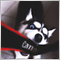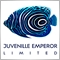# I need help in creating an expert . EXPERT WITH INDICATOR AUTO FIBONACCI LEVELS11

### How need to work : Indcator show a sell position or a buy position . Ea need to set Instant execution on fibonacci levels .If price hit one level in fibonacci indicator , the ea  set instant execution , for example if price hit 62 level , ea check the indicator position and know that it is a buy or a sell , and set instiant execution ( 62 level ) tp ( on 38 level )  and sl (on 100 level )  ( check photos down ) . If trade hit TP1 ,ea will be set SL on entry . If indicator change or show another direction , Ea will be close all position .

____________________________________________________________________________________________________________________________________________

Inputs Paramets for EA

____________________________________________________________________________________________________________________________________________

Trade Lot Size = 0.01  ( you can choice your risk )

Alert Entry = True/False ( you can set a alert if EA set a trade . Alert show on what Fibonacci level is a entry (62 or 70 or 79) and on what simbol (eurusd,gbp..))

Entry on Fibonacci level 0.62 = True/False  ( you can choice if you want EA to set on that Fibonacci level trades )

Entry on Fibonacci level 0.70 = True/False  ( you can choice if you want EA to set on that Fibonacci level trades )

Entry on Fibonacci level 0.79 = True/False  ( you can choice if you want EA to set on that Fibonacci level trades )

TakeProfit on Fibonacci level = 0.23%   ( you can choice if you want to set tp on that level or another Fibonacci indicator level for example on 0.23 or 0.38,0.0,100)

TakeProfit on Fibonacci level = 0.38%    ( you can choice if you want to set tp on that level or another Fibonacci indicator level for example on 0.23 or 0.38,0.0,100)

TakeProfit on Fibonacci level = 0.00%    ( you can choice if you want to set tp on that level or another Fibonacci indicator level for example on 0.23 or 0.38,0.0,100)

TakeProfit on Fibonacci level = 100.0%  ( you can choice if you want to set tp on that level or another Fibonacci indicator level for example on 0.23 or0.38,0.0,100)

Aotomatical set StopLoss on entry = True/False   ( you can choice if you want to set SL on entry if trade hit TP 1 for others trades )

____________________________________________________________________________________________________________________________________________

If you know an EA who work like that plan , please conntact me , If you can make it conntact me m if you need more detailes conntact me .

That Indicator Auto Fibonacci levels work on all timeframes and on all simbols , EA need to work the same on all timeframe and all simbols

Files: